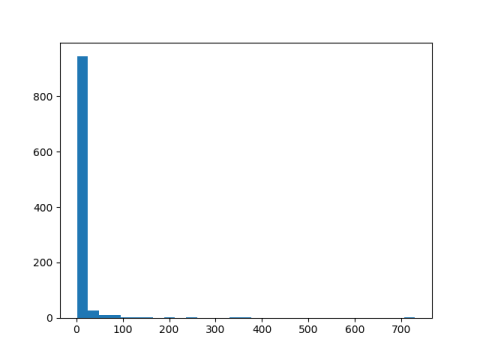# Hadamard’s upper bound on determinant

For an n by n real matrix A, Hadamard’s upper bound on determinant iswhere aij is the element in row i and column j. See, for example, .

How tight is this upper bound? To find out, let’s write a little Python code to generate random matrices and compare their determinants to Hadamard’s bounds. We’ll take the square root of both sides of Hadamard’s inequality to get an upper bound on the absolute value of the determinant.

Hadamard’s inequality is homogeneous: multiplying the matrix A by λ multiplies both sides by λn. We’ll look at the ratio of Hadamard’s bound to the exact determinant. This has the same effect as generating matrices to have a fixed determinant value, such as 1.


from scipy.stats import norm
from scipy.linalg import det
import matplotlib.pyplot as plt
import numpy as np

# Hadamard's upper bound on determinant squared
return np.prod(np.sum(A**2, axis=1))

N = 1000
ratios = np.empty(N)

dim = 3
for i in range(N):
A = norm.rvs(size=(dim, dim))

plt.hist(ratios, bins=int(N**0.5))
plt.show()



In this simulation the ratio is very often around 25 or less, but occasionally much larger, 730 in this example.It makes sense that the ratio could be large; in theory the ratio could be infinite because the determinant could be zero. The error is frequently much smaller than the histogram might imply since a lot of small values are binned together.

I modified the code above to print quantiles and ran it again.

    print(min(ratios), max(ratios))
qs = [0.05, 0.25, 0.5, 0.75, 0.95]
print( [np.quantile(ratios, q) for q in qs] )


This printed

    1.0022 1624.9836
[1.1558, 1.6450, 2.6048, 5.7189, 32.49279]


So while the maximum ratio was 1624, the ratio was less than 2.6048 half the time, and less than 5.7189 three quarters of the time.

Hadamard’s upper bound can be very inaccurate; there’s no limit on the relative error, though you could bound the absolute error in terms of the norm of the matrix. However, very often the relative error is moderately small.